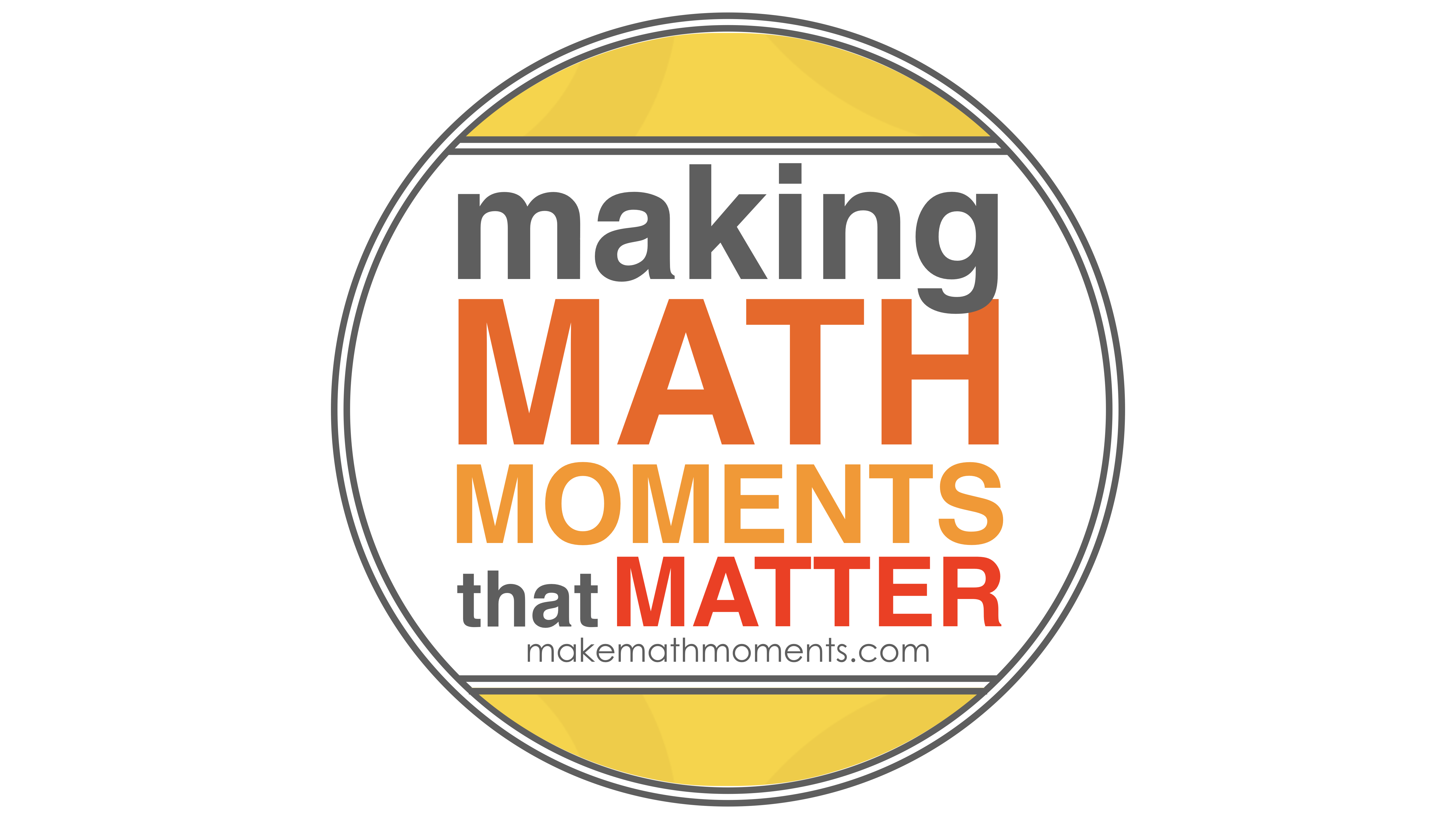# Category - Number Sense

## Magnitude Of Number: Where Does 1 Billion Go?

Explore magnitude of number by trying to determine where 1 billion should go on a number line from 0 to 1 trillion based on a Mark Chubb Tweet.

## Solving Two-Step Equations Visually Part 2

In this series of Math Is Visual Prompts, we slowly introduce the idea of Solving Two-Step Equations Using Partitive and Quotative Division Scenarios.

## Solving Two-Step Equations Visually

In this series of Math Is Visual Prompts, we slowly introduce the idea of Solving Two-Step Equations Using Partitive Division Scenarios.

## Solving One-Step Equations Visually

In this series of Math Is Visual Prompts, we slowly introduce the idea of Solving One-Step Equations Using Partitive and Quotative Division.

## Comparing and Ordering On A Number Path

In this series of Math Is Visual Prompts, we slowly introduce the idea of a linear model (i.e.: number line) by placing numbers on the number path.

## Using Number Paths As A Linear Model

In this series of Math Is Visual Prompts, we slowly introduce the idea of a linear model (i.e.: number line) through the use of a number path. This...

## Multiplication Number Talk – Unpacking Halving and Doubling Using Number Properties

Explore a multiplication number talk that explicitly unpacks the doubling and halving strategy to show how number properties like commutative &...

## Visualizing Early Addition and Subtraction Question Structures

In this Math Is Visual video animation and visual prompts, we will explore early addition and subtraction question structures through concrete and...

## Visualizing Integer Division: Positive Divided By a Negative

In this Math Is Visual video animation and visual prompts, we will explore negative divided by a positive integer division conceptually through...

## Visualizing Integer Division: Negative Divided By a Positive

In this Math Is Visual video animation and visual prompts, we will explore integer division through quotative division and partitive division! In...

GET MATH IS VISUAL PROMPTS TO YOUR INBOX!